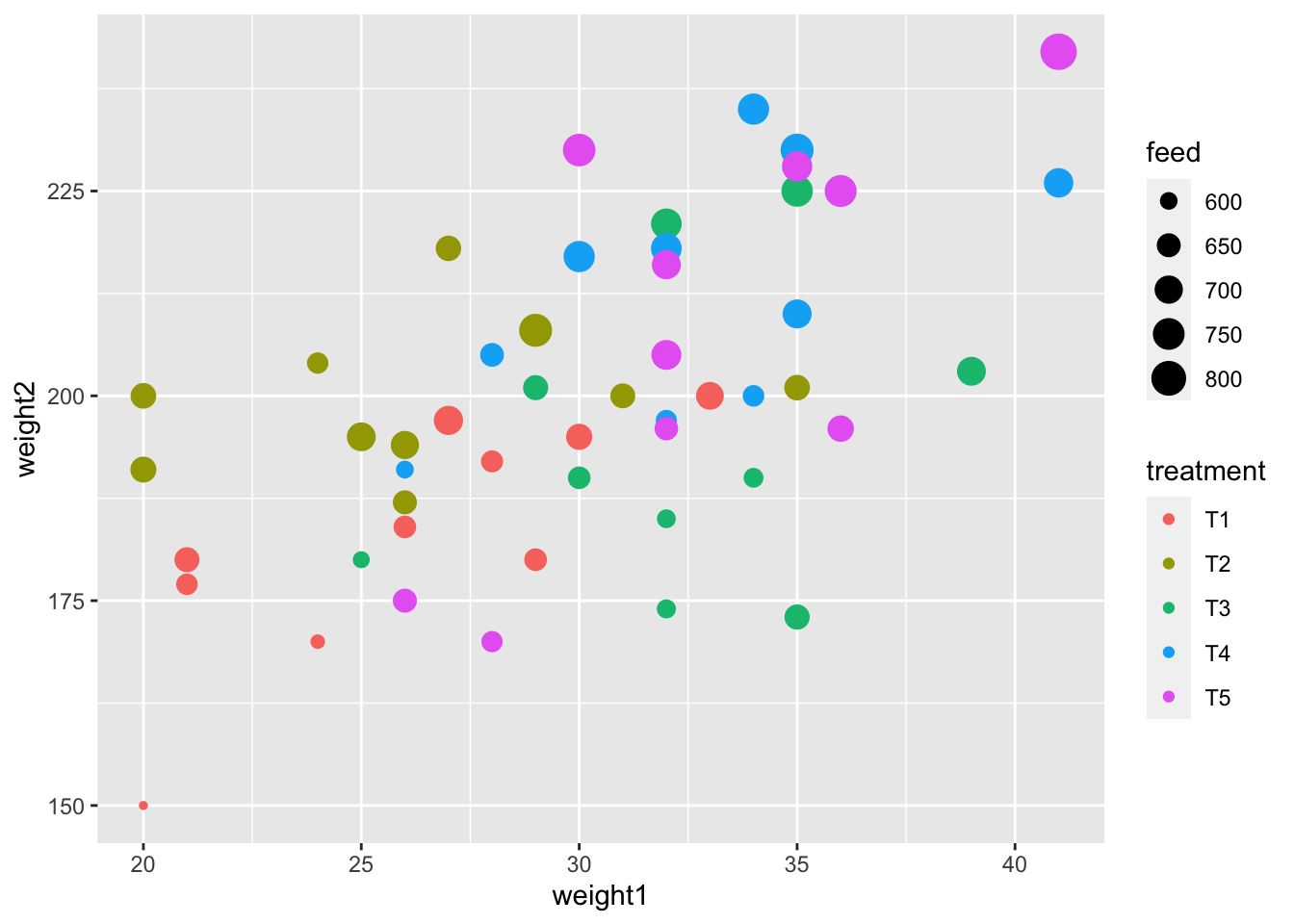## Exercise 1.1: Scatterplot

``````ggplot(crampton.pig, aes(weight1, weight2, color = treatment, size = feed)) +
geom_point()``````## Exercise 1.2: Tile plot

``````ggplot(crampton.pig, aes(rep, treatment, fill = weight2 - weight1)) +
geom_tile(color = "black", size = 2)``````